Next: Single bisection mode Up: Mathematical background Previous: Using the monotonicity   Contents

Improving the evaluation using the Jacobian and centered form

Let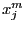be the middle point of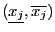and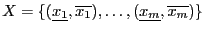be the box. Then: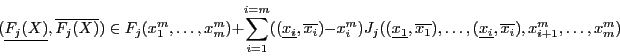(2.4)

see , pp. 52. This expression enable to get in some cases a sharper bound on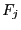.

This evaluation is embedded into the evaluation procedure of the solving algorithms using the Jacobian. It is also available in its general form as

INTERVAL_VECTOR Centered_Form(int DimVar,int DimEq,
INTERVAL_VECTOR (* TheIntervalFunction)(int,int,INTERVAL_VECTOR &),
INTERVAL_MATRIX (* Gradient)(int, int, INTERVAL_VECTOR &),
VECTOR &Center,
INTERVAL_VECTOR &Input)
where
• DimVar: number of variables
• DimEq: number of expressions
• TheIntervalFunction: procedure in MakeF format for interval evaluating the expressions
• Gradient: procedure un MakeJ format for evaluating the derivatives of the expressions
• Center:the center point for the centered form
• Input: the ranges for the variables
A variant of this procedure is

INTERVAL Centered_Form(int k,int DimVar,int DimEq,
INTERVAL_VECTOR (* TheIntervalFunction)(int,int,INTERVAL_VECTOR &),
INTERVAL_MATRIX (* Gradient)(int, int, INTERVAL_VECTOR &),
VECTOR &Center,
INTERVAL_VECTOR &Input)
which is used to evaluate only expression number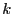.

A more sophisticated evaluation for the centered form is based on Baumann theorem . First we define the procedure cut(double x,INTERVAL X) as: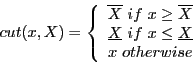For a system of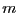equations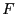inunknowns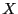we define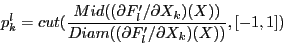For a given equation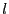we use the centered form with as center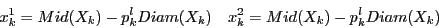within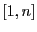. The choice for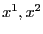is based on the property that the lower end-point of the centered form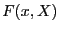has a maximum at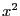while its upper end-point has a minimum at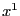. The interval evaluation of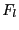is obtained as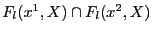. Although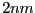centered form are used to compute the interval evaluation of theequations the calculation is in fact not so expensive as the interval evaluation of the Jacobian matrix has to be done only once.

The implementation is:

INTERVAL_VECTOR BiCentered_Form(int DimVar,
int DimEq,
INTERVAL_VECTOR (* TheIntervalFunction)(int,int,INTERVAL_VECTOR &),
INTERVAL_MATRIX (* Gradient)(int, int, INTERVAL_VECTOR &),
INTERVAL_VECTOR &Input,
int Exact)
where
• DimVar: number of variables
• DimEq: number of expressions
• TheIntervalFunction: procedure in MakeF format for interval evaluating the expressions
• Gradient: procedure un MakeJ format for evaluating the derivatives of the expressions
• Input: the ranges for the variables
• Exact: if 0 the procedure will return as soon as an interval evaluation of one expression does not include 0
A variant for evaluating only equation numberis

INTERVAL_VECTOR BiCentered_Form(int k,int DimVar,
int DimEq,
INTERVAL_VECTOR (* TheIntervalFunction)(int,int,INTERVAL_VECTOR &),
INTERVAL_MATRIX (* Gradient)(int, int, INTERVAL_VECTOR &),
INTERVAL_VECTOR &Input,
int Exact)

Another variant is based on the property that the numerical interval evaluation of the product J(Input)(Input-Center) may be overestimated as there may be several occurence of the same variable in this product. We may assume that this product has been computed symbolically, then re-arranged to reduce the number of occurence of the same variable leading to a procedure in MakeF format that computes directly the product. The syntax of the bicentered form procedure is

INTERVAL_VECTOR BiCentered_Form(int DimVar,
int DimEq,
INTERVAL_VECTOR (* TheIntervalFunction)(int,int,INTERVAL_VECTOR &),
INTERVAL_MATRIX (* Gradient)(int, int, INTERVAL_VECTOR &),
INTERVAL_VECTOR (* ProdGradient)(int, int, INTERVAL_VECTOR &),
INTERVAL_VECTOR &Input,
int Exact)
where ProdGradient is the procedure that computes the product J(Input)(Input-Center), being understood that the Center is available in the global variable ALIAS_Center_CenteredForm.Next: Single bisection mode Up: Mathematical background Previous: Using the monotonicity   Contents
Jean-Pierre Merlet 2012-12-20